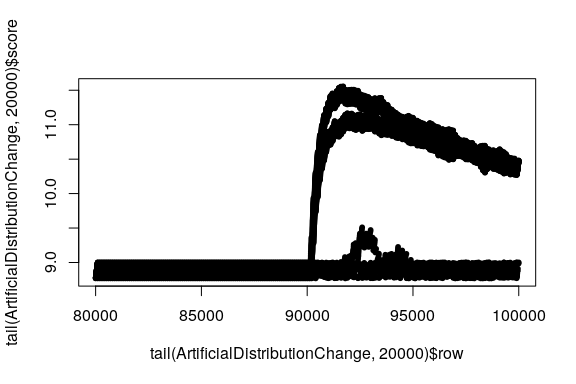# Introduction

‘MIDAS’ is a fast and state of the art approach of anomaly detection in edge-based graphs. In this short introduction an inside on how to use this wrapper of the C++ implementation of ‘MIDAS’ and ‘MIDAS-R’ (Bhatia et al., 2020) by Siddharth Bhatia is given.

There are two example datasets given by this package, ‘MIDASexample’ originally included in the original C++ implementation and an artifical dataset ‘ArtificialDistributionChange’ with a sudden change of edge distribution around times = 900 (row number: 90.000).

In this example we’re working with the artificial dataset. It’s not necessarily a representative network structure containing one node (source = 1) connecting to several other nodes (destination = 2-20), but it should serve well for demonstration purposes.

## Basic example

First we load the library and the dataset ‘ArtificialDistributionChange’ into the R environment. The structure of the dataset is based on the ‘data.frame’ structure needed as an input to the ‘MIDAS’ scoring function. src (‘source’) and dst (‘destination’) describing an edge of the network at ‘timestamp’ times. All provided datatypes must be integers.

library(MIDASwrappeR)
data("ArtificialDistributionChange")
#>   src dst times
#> 1   1   2     1
#> 2   1   2     1
#> 3   1   5     1
#> 4   1   2     1
#> 5   1   3     1
#> 6   1   3     1
ArtificialDistributionChange$row <- 1:nrow(ArtificialDistributionChange) The distribution before and after the change of the network structure is shown in these histograms: hist(subset(ArtificialDistributionChange,ArtificialDistributionChange$row<90000)$dst,freq=F)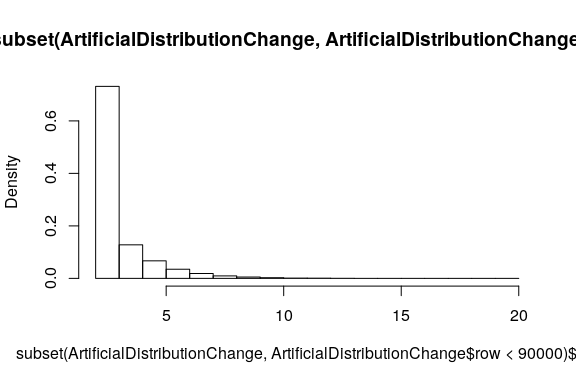hist(subset(ArtificialDistributionChange,ArtificialDistributionChange$row>=90000)$dst,freq=F)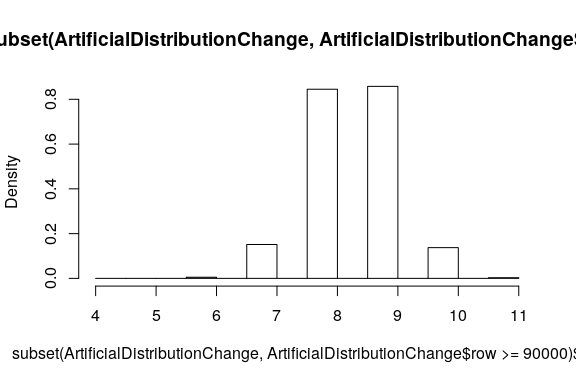We see several nodes disappearing and some nodes are connected significantly more often (destination: 7,8). The base structure of some nodes is still preserved. ## Scoring The ‘data.frame’ is as is ready to compute the scoring: ArtificialDistributionChange$score <- getMIDASScore(ArtificialDistributionChange)

plot(x=tail(ArtificialDistributionChange, 20000)$row, y=tail(ArtificialDistributionChange, 20000)$score, pch=20)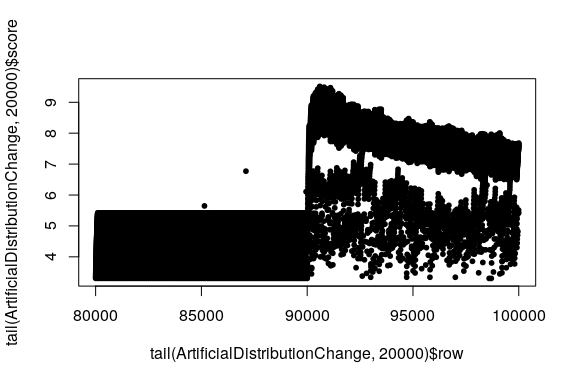We see a sudden increase in score at row 90.000 which indicates abnormalities in the structure of the network. We can further specifically check the nodes responsible for the sudden change of score:

head(subset(ArtificialDistributionChange, ArtificialDistributionChange$score > 8)) #> src dst times row score #> 90088 1 9 901 90088 8.025332 #> 90090 1 9 901 90090 8.070556 #> 90092 1 9 901 90092 8.114697 #> 90094 1 9 901 90094 8.157804 #> 90095 1 9 901 90095 8.199924 #> 90099 1 9 901 90099 8.241099 The underlying change of distribution is detected by the algorithm shortly after the change at row number 90.000. ## Parameters ### undirected The algorithm can handle both directed and undirected graph edges. In case of an undirected graph as an input no transformation needs to be done outside of the scoring function. Internally the ‘data.frame’ is doubled in size and the result will be doubled as well. A simple boolean masking operator leads to the expected number of rows. ArtificialDistributionChange$score <- getMIDASScore(ArtificialDistributionChange, undirected=T)[c( F, T )]
plot(x=tail(ArtificialDistributionChange, 20000)$row, y=tail(ArtificialDistributionChange, 20000)$score, pch=20)## norelations

By default ‘MIDAS-R’ is used to describe edges in a temporal manner keeping track of temporal and spatial proximity. If this behaviour is not needed this behaviour can be turned off:

ArtificialDistributionChange$score <- getMIDASScore(ArtificialDistributionChange, norelations=T) plot(x=tail(ArtificialDistributionChange, 20000)$row, y=tail(ArtificialDistributionChange, 20000)$score, pch=20)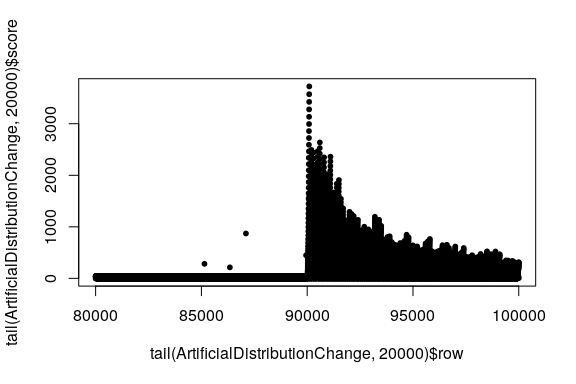As shown in this example: This can provide a clearer signal (baseline 10 to peak 3700 vs. 4 to 9) in some cases, at a loss of spatial-temporal analytic capabilities. ## alpha In ‘MIDAS-R’ edges will be analyzed not only spatially, edges in the recent past should also count toward the current time tick, but modified by a reduced weight. Every time tick - as given by our ‘data.frame’ column times - the effect of previous structures is reduced by a fraction alpha. This parameter has no effect, when ‘MIDAS’ instead of ‘MIDAS-R’ is used. Let’s try a much higher and a much lower value than the default value of 0.6: ArtificialDistributionChange$score <- getMIDASScore(ArtificialDistributionChange, alpha = .9)
plot(x=tail(ArtificialDistributionChange, 20000)$row, y=tail(ArtificialDistributionChange, 20000)$score, pch=20)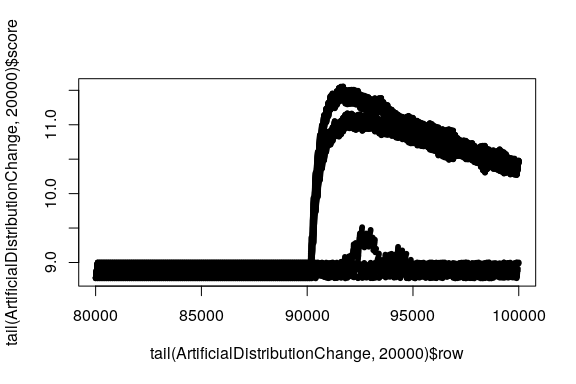The increase of alpha reduced noise and the distinction between edges that could be found in the base graph and edges that show strange odd behaviour is much easier.

aggregate(score ~ dst, data=subset(ArtificialDistributionChange,ArtificialDistributionChange$times==907) , max) #> dst score #> 1 7 8.989773 #> 2 8 10.564036 #> 3 9 10.996455 #> 4 10 8.996424 #> 5 11 8.998636 Unsurprisingly, nodes 8 and 9 have especially high scores while the nodes 7, 10 & 11 show minor, but still visible unregularities as shown on the graph. ArtificialDistributionChange$score <- getMIDASScore(ArtificialDistributionChange, alpha = .1)
plot(x=tail(ArtificialDistributionChange, 20000)$row, y=tail(ArtificialDistributionChange, 20000)$score, pch=20)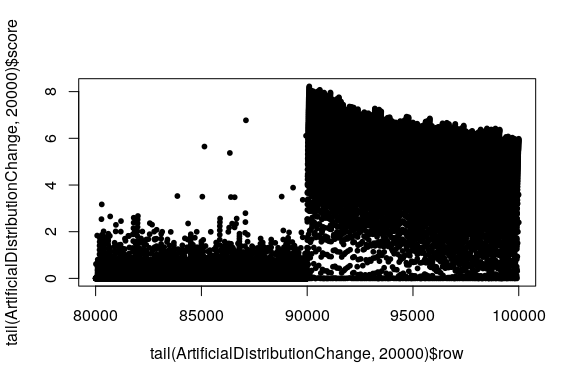Whereas, unsurprisingly, a lower alpha value increased the noise significantly. An optimal value for alpha should be evaluated based on the input data.

## buckets & rows

In terms of memory, both ‘MIDAS’ and ‘MIDAS-R’ only need to maintain the data structures over time, which are proportional to O(wb), where w and b are the number of hash functions and the number of buckets in the CMS data structures; which is bounded with respect to the data size as stated in the original paper. There values should be choosen based upon the input data. Other than that in some cases it could lead to interesting experimental insights on the underlying data:

ArtificialDistributionChange$score <- getMIDASScore(ArtificialDistributionChange, alpha = .9, buckets=10) plot(x=tail(ArtificialDistributionChange, 20000)$row, y=tail(ArtificialDistributionChange, 20000)\$score, pch=20)